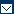## Methods of DepreciationTweet (function() { var po = document.createElement('script'); po.type = 'text/javascript'; po.async = true; po.src = 'https://apis.google.com/js/plusone.js'; var s = document.getElementsByTagName('script'); s.parentNode.insertBefore(po, s); })();

The fall in an asset’s value is known as depreciation. It is for a fixed period and is considered an expense. The value has to be added in expenses of that period, as it has a direct impact on profit/loss. In the same way, the amount is subtracted from the asset’s value to reach book value for every year.

As per the IFRS, an organization must select a depreciation policy at the start of the business. However, it also allows the business to change its policies, if there is a genuine reason.

There are several methods of depreciation. Generally, businesses choose the one that syncs well with the nature of the assets.

Some very commonly used methods of depreciation are:

## Straight-line Depreciation

The simplest and most commonly used depreciation method is the straight-line method. In this method, the company estimates the asset’s salvage value (resale value when the company decides to sell it), which is subtracted from the original value of the asset.  Salvage value may be zero or in negative.

After subtracting the salvage value, the remaining amount is divided by the useful life of asset, as clearly shown in the formula below:The main reason for the success of this method is the fact that it is very easy to compute. However, due to the ambiguous nature of the estimate, some companies do not consider it the right option.

## Declining Balance Method

This method gives a higher depreciation charge in the first year, as the balance keeps on declining every year. This method is a more realistic approach, as assets are most useful when they are new. It is understood that the value declines with time.

However, an upgrade might increase the value of an asset. This method of depreciation allows accountants to re-evaluate the value of an asset, if the requirement meets the criteria set by the IFRS.

## Sum-of-the-years’ Digits Method

In this method, the depreciation value is multiplied by a schedule of fractions to determine the annual depreciation amount. Just like declining balance method, the value of the asset keeps on decreasing every year (with a different percentage), and finally reaches a salvage value, where it is disposed off or sold in the market.

Formula: Depreciable Cost = Original Cost − Salvage Value

Formula: Book Value = Original Cost − Accumulated Depreciation

There are a few other methods of depreciation too such as:

(1) Units-of-production method

(2) Units-of-time method

(3) Group depreciation method.

However, the above mentioned methods are the most commonly used methods by most accountants.Contact Sitemap Links Copyright 2022 Best-Practice.com. All Rights Reserved.